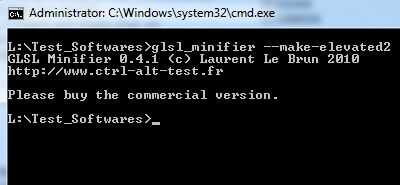# [3D Programming] GLSL Minifier: Pack Your GLSL Code Into a C HeaderGLSL Minifier is a tool made by a french demogroup (yeah!) to pack GLSL shader code into a C header file. It generates a code as small as possible that is equivalent to the input code. GLSL Minifier has been optimized for the compression tool Crinkler.

See this post for some tips to create very small code and help compression tools like Crinkler.

I tested GLSL Minifier with the following pixel shader saved in a file called ps.glsl:

```#version 120
uniform sampler2D blurTex;
uniform float rt_w;
uniform float rt_h;
// Kernel weight vector
float samples;

uniform float kernel;

vec4 BlurV(vec2 Tex)
{
vec4 Color = vec4(0.0);
for (int i = 0; i<5; i++)
Color += texture2D(blurTex,
vec2(Tex.x, Tex.y +  samples[i])
) * kernel[i];
return Color;
}

void main()
{
vec2 uv = gl_TexCoord.xy;
samples = -4.0/rt_w;
samples = -2.0/rt_w;
samples = 0.0;
samples = 2.0/rt_w;
samples = 4.0/rt_w;
gl_FragColor = BlurV(uv);
}```

I ran GLSL_Minifier in command line:

```glsl_minifier ps.glsl
```

and here is the C file generated by GLSL_Minifier:

```/* File generated with GLSL Minifier 0.4.1
* http://www.ctrl-alt-test.fr
*/
#define U_KERNEL "t"
#define U_RT_H "e"
#define U_RT_W "o"
#define U_BLURTEX "r"
"#version 120\n"
"uniform sampler2D r;"
"uniform float o,e;"
"float v;"
"uniform float t;"
"vec4 n(vec2 o)"
"{"
"vec4 e=vec4(0.);"
"for(int n=0;n<5;n++)"
"e+=texture2D(r,vec2(o.r,o.g+v[n]))*t[n];"
"return e;"
"}"
"void main()"
"{"
"vec2 r=gl_TexCoord.rg;"
"v=-4./o;"
"v=-2./o;"
"v=0.;"
"v=2./o;"
"v=4./o;"
"gl_FragColor=n(r);"
"}";
```

GLSL Minifier has an option called --make-elevated2. Here is the output 😀[via]

## 6 thoughts on “[3D Programming] GLSL Minifier: Pack Your GLSL Code Into a C Header”

1.jammer

looks like useful tool 🙂

2.ca\$per

Call me stupid, but i just don’t get it. Why is this conversion needed? Where are those defined used? What is this for? The only thing i can think of is demo making, to place all resources in EXE file. But it’s not hard to copy/paste a shader code to *.c file.
Just don’t get it… Can someone explain it to me?

3.sqrt[-1]

When making a 1K or 4K demo that embeds a shader in the exe, you need to strip out as much as possible to fit the size.

This tool allows you to write the shaders normally and then strip all the excess syntax that is not needed.

4.LLB

ca\$per: this tool was made specifically for the demos. When you want to use complex shaders in a 1k or 4k intro, it requires a lot of work to make them as small as possible – especially if you consider the code will be compressed (e.g. there are many tricks to use in the variables names). It might also be used as a GLSL obfuscator.

JeGX, thanks for the article!

5.JeGX Post Author

@LLB: pas de probleme Laurent, dès qu’un outil pour la 3D est cool je le mets en avant, d’autant plus qu’il est francophone 😉

6.ca\$per

Hmm… I get it now.
Thanks guys!